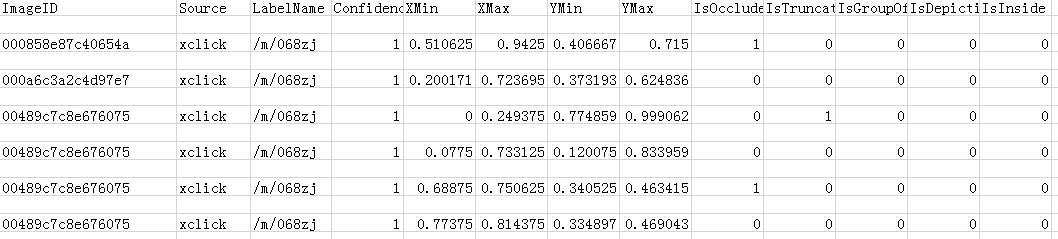• 2020-12-01 19:11:31

python读取csv的文件，可以用pandas包来读取，比如

import pandas as pd
print(df)


读取数据后，若要获取某一行某一列的值，

# 获取某一行的值
print(df.iloc)

# 获取某一列的值
print(df["item_id"]) # 列名是item_id的列

# 获取某一行某一列的值
print(df.iloc["item_id"])

更多相关内容
• 读取CSV文件,把csv文件放在份list中。 ''' import csv class readCSV(object): def __init__(self,path="Demo.csv"): #创建个属性用来保存要操作CSV的文件 self.path=path try: #打开个csv文件，并赋予读...
• 现有如图的csv文件，已知deck的部分值，想取根据对应deck的rate值。
• ## Python读取csv的某列

千次阅读 2020-11-30 11:00:19
CSV 本质上是文本文件，能提供与 Excel 的...本文讨论如何快速有效的按列读取 CSV 文件。假设“A.csv“文件内容是：No.,Name,Age,Score1,Apple,12,982,Ben,13,973,Celia,14,964,Dave,15,95用 Excel 打开这个 csv， ...CSV 本质上是文本文件，能提供与 Excel 的表格类似的二维数据结构。因为是文本文件，读写都比 Excel 方便容易的多，经常拿来作为数据的中转格式。本文讨论如何快速有效的按列读取 CSV 文件。

假设“A.csv“文件内容是：

No.,Name,Age,Score

1,Apple,12,98

2,Ben,13,97

3,Celia,14,96

4,Dave,15,95

用 Excel 打开这个 csv， 看起来是这样的：利用Python自带的 csv 模块 ，有两种方法可以提取其中的一列：

import csv

with open('A.csv','rb') as csvfile:

rows= [row for row in reader]

print rows

得到：

[['No.', 'Name', 'Age', 'Score'],

['1', 'Apple', '12', '98'],

['2', 'Ben', '13', '97'],

['3', 'Celia', '14', '96'],

['4', 'Dave', '15', '95']]

要提取其中某一列，可以用下面的代码：

import csv

with open('A.csv','rb') as csvfile:

column = [row for row in reader]

print column

得到：

['Age', '12', '13', '14', '15']

import csv

with open('A.csv','rb') as csvfile:

column = [row for row in reader]

print column

得到：

[{'Age': '12', 'No.': '1', 'Score': '98', 'Name': 'Apple'},

{'Age': '13', 'No.': '2', 'Score': '97', 'Name': 'Ben'},

{'Age': '14', 'No.': '3', 'Score': '96', 'Name': 'Celia'},

{'Age': '15', 'No.': '4', 'Score': '95', 'Name': 'Dave'}]

import csv

with open('A.csv','rb') as csvfile:

column = [row['Age'] for row in reader]

print column

就得到：

['12', '13', '14', '15']

展开全文• 下面小编就为大家分享篇使用python获取csv文本的行或某列数据的实例，具有很好的参考价值，希望对大家有所帮助。一起跟随小编过来看看吧
• ## Python读取csv文件中的某一列

万次阅读 多人点赞 2020-02-12 15:18:23
比如这样的csv文件： 想要读取imageID和label 注：imageID的第数据是：000858e87c40654a ...data = pd.read_csv(path) #读取文件中所有数据 # 按分离数据 x = data[['ImageID', 'label']]#读取...

比如这样的一个csv文件：
想要读取imageID和label注：imageID的第一个数据是：000858e87c40654a

import pandas as pd
path = 'yourfile.csv'
# 使用pandas读入
# 按列分离数据
x = data[['ImageID', 'label']]#读取某两列
print(x)
y = data[['ImageID']]#读取某一列
print(y)


如果只读取一列数据，可以直接用y[i]访问

print(type(y)) #<class 'pandas.core.series.Series'>
print(len(y)) #1637
print(y)	#000858e87c40654a
print(type(y)) #str
print('000858e87c40654a' in y) #false

展开全文• path_csv='C:\\Users\\11982\\feiyan\\train_bboxes.csv' data=pd.read_csv(path_csv) data['filename']=data['filename'].astype(str) data['filename']=data['filename'].apply(lambda x:r'.\\feiyan\\train\\'+x+...

import pandas as pd
path_csv='C:\\Users\\11982\\feiyan\\train_bboxes.csv'
data['filename']=data['filename'].astype(str)
data['filename']=data['filename'].apply(lambda x:r'.\\feiyan\\train\\'+x+'.jpg')
data.to_csv(path_csv)

index=false表示不加索引

import os
import shutil
import pandas as pd
path_csv='C:\\Users\\11982\\feiyan\\train_bboxes.csv'
# data['filename']=data['filename'].astype(str)
# data['filename']=data['filename'].apply(lambda x:x+'.jpg')
# data.to_csv(path_csv)
path_img='C:\\Users\\11982\\feiyan\\train'
ls = os.listdir(path_img)
# # print(len(ls))
ans={}
for i in range(len(data['filename'])):
for j in range(len(data['filename'])):
if str(data['filename'][i])==str(ls[j]):
print(data['filename'][i])
#             ans[i]=data['filename'][i]
#             shutil.move(path_img+'\\'+ls[j],"C:\\Users\\11982\\feiyan\\annotation\\"+ls[j])
shutil.copy(path_img+'\\'+ls[j],"C:\\Users\\11982\\feiyan\\annotation\\"+ls[j])
print(ls[j])


import os
import shutil
import pandas as pd
new=[]
path_csv='C:\\Users\\11982\\feiyan\\1.csv'
# data['filename']=data['filename'].astype(str)
# data['filename']=data['filename'].apply(lambda x:x+'.jpg')
# data.to_csv(path_csv)
path_img='C:\\Users\\11982\\feiyan\\train.csv'
# # print(len(ls))
ans={}
for i in range(len(data['filename'])):
for j in range(len(ls['0'])):
if str(data['filename'][i])==str(ls['0'][j]):
print(data['filename'][i])
new.append(ls['1'][j])
data['nums']=new
data.to_csv(path_csv,index=False)

展开全文• 您可以尝试使用pandas，它为处理数据提供了个很好的结构。在将csv读到DataFrameIn : import pandas as pdIn : df = pd.read_csv('test.csv', skipinitialspace=True)In : dfOut:timeStamp ...
• Python 读取csv行 转载 2016年08月30日 21:01:44 标签： python / csv / 数据 站长用Python写了个可以提取csv任一的代码，欢迎使用。Github链接 csv是Comma-Separated Values的缩写，...
• pandas获取csv指定行，列 house_info = pd.read_csv('house_info.csv') 1：取行的操作： house_info.loc[3:6]类似于python的切片操作 2：取列操作： house_info['price'] 这是读取csv...5：对某一列除以他的最大值，
• 我有csv文件，如下所示：+-----+-----+-----+-----+-----+-----+-----+-----+| AAA | bbb | ccc | DDD | eee | FFF | GGG | hhh |+-----+-----+-----+-----+-----+-----+-----+-----+| 1 | 2 | 3 | 4 | 50 | 3 |...
• （缺点：有缺失值就无法读取） 读： import numpy my_matrix = numpy.loadtxt(open("1.csv","rb"),delimiter=",",skiprows=0) 写： numpy.savetxt('2.csv', my_matrix, delimiter = ',') 可能遇到的问题： ...
• 有的时候我们把数据整理收集整理到excel中，那么要把excel中的某列文本数据制作词云图怎么做呢？ 从最简单的说起。 最简单的词云图就是这种，英文的，不读取文件，不指定背景形状图片，不指定字体。 #复制文本用字符...
• 今天小编就为大家分享python pandas读取csv后,获取标签的方法，具有很好的参考价值，希望对大家有所帮助。一起跟随小编过来看看吧
• 请问大家，我现在可以读出csv文件中某一列数据，但是我现在想把它提取出来并且使其成为一个新的列表，然后对其其中仅有的三种情况分别赋值，可是我现在不知道怎么把特定列的内容生成为一个列表，谁有办法吗？...
•list
• 我使用pythonCSV模块遍历的行。我需要做的是：获取“title”的第行移除任何西班牙文字符(重音，Ñ)删除单引号最后，用破折号替换空格，并将所有内容转换为小写。我得到这个工作与个简单的测试文件，而不是...
• csv是Comma-Separated Values的缩写，是用文本文件形式储存的表格数据，比如如下的表格：就可以存储为csv文件，文件内容是：No.,Name,Age,...如何用Python像操作Excel一样提取其中的一列，即一个字段，利用Python...
• 在学习python过程中，遇到种情况，要读取.csv文件的数据，然后取出其中某个字段，然后再写入到个文件中，用于数据的比对，代码如下： import csv import hashlib from itertools import islice from openpyxl ...
• 给定.csv文件温度csv在L1x,,,,,,,0,1,0,0,0,1,1,0,0,1,0,0,1,0,0,0,0,1,0,0,1,0,0,0,0,1,0,0,1,1,1,1,1,1,1,1,1,1,1,1,1,1,1,1,1,1,1,1,1,1,1,1,1,1,1,1,读入如下：^{pr2}\$输出：c0 c1 c2 c3 c4 c5 c6 c70 0 1 0 0 ....
• 使用python的pandas库读取某几列CSV文件，再写入新的文件。 ''' import pandas as pd import time #处理函数 def readwrite(input_file,output_file): #pd.read_csv() 将CSV文件读入并转化为数据框(DataFrame)...
• 我对Python还很陌生，我编写了一个脚本来解析...如果我在末尾添加另一列，即使是空列，它也会打印出前一列中的数据，因此该列的内容不会有问题。在代码的删节版(但仍然很暴躁)：import osos.chdir('C:\\Python34\\a...
• wd = pd.read_csv('cp.csv') word = [i for i in wd[['关键词']].values] value = [i for i in wd[['词频']].values] wordCloud = WordCloud(width=2000, height=2000) wordCloud.add('词云', word, value, ......